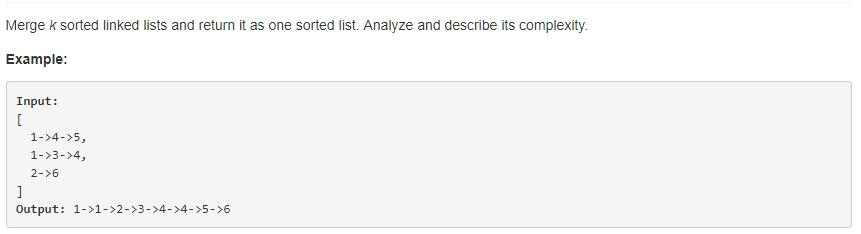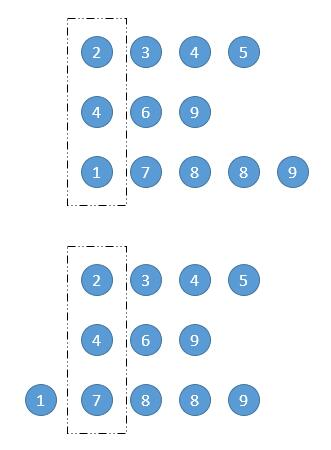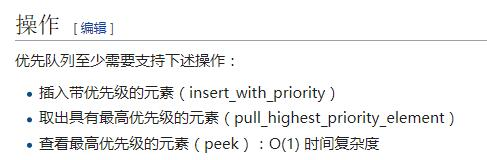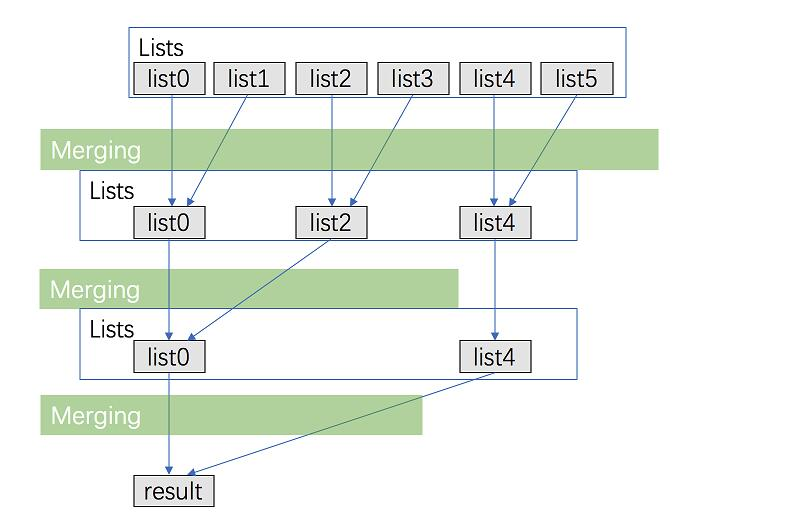## 1. 题目描述（困难难度）k 个有序链表的合并。

# 解法一 暴力破解

public ListNode mergeKLists(ListNode[] lists) {
List<Integer> l = new ArrayList<Integer>();
//存到数组
for (ListNode ln : lists) {
while (ln != null) {
ln = ln.next;
}
}
//数组排序
Collections.sort(l);
//存到链表
for (int i : l) {
ListNode t = new ListNode(i);
h.next = t;
h = h.next;
}
h.next = null;
}


# 解法二 一列一列比较public ListNode mergeKLists(ListNode[] lists) {
int min_index = 0;
while (true) {
boolean isBreak = true;//标记是否遍历完所有链表
int min = Integer.MAX_VALUE;
for (int i = 0; i < lists.length; i++) {
if (lists[i] != null) {
//找出最小下标
if (lists[i].val < min) {
min_index = i;
min = lists[i].val;
}
//存在一个链表不为空，标记改完 false
isBreak = false;
}

}
if (isBreak) {
break;
}
//加到新链表中
ListNode a = new ListNode(lists[min_index].val);
h.next = a;
h = h.next;
//链表后移一个元素
lists[min_index] = lists[min_index].next;
}
h.next = null;
}


public ListNode mergeKLists(ListNode[] lists) {
int min_index = 0;
while (true) {
boolean isBreak = true;
int min = Integer.MAX_VALUE;
for (int i = 0; i < lists.length; i++) {
if (lists[i] != null) {
if (lists[i].val < min) {
min_index = i;
min = lists[i].val;
}
isBreak = false;
}

}
if (isBreak) {
break;
}
//最小的节点接过来
h.next = lists[min_index];
h = h.next;
lists[min_index] = lists[min_index].next;
}
h.next = null;
}


# 解法三 优先队列public ListNode mergeKLists(ListNode[] lists) {
//定义优先队列的比较器
Comparator<ListNode> cmp;
cmp = new Comparator<ListNode>() {
@Override
public int compare(ListNode o1, ListNode o2) {
// TODO Auto-generated method stub
return o1.val-o2.val;
}
};

//建立队列
Queue<ListNode> q = new PriorityQueue<ListNode>(cmp);
for(ListNode l : lists){
if(l!=null){
}
}
while(!q.isEmpty()){
//出队列
point.next = q.poll();
point = point.next;
//判断当前链表是否为空，不为空就将新元素入队
ListNode next = point.next;
if(next!=null){
}
}
}


# 解法四 两两合并

public ListNode mergeTwoLists(ListNode l1, ListNode l2) {
ListNode h = new ListNode(0);
ListNode ans=h;
while (l1 != null && l2 != null) {
if (l1.val < l2.val) {
h.next = l1;
h = h.next;
l1 = l1.next;
} else {
h.next = l2;
h = h.next;
l2 = l2.next;
}
}
if(l1==null){
h.next=l2;
}
if(l2==null){
h.next=l1;
}
return ans.next;
}
public ListNode mergeKLists(ListNode[] lists) {
if(lists.length==1){
return lists;
}
if(lists.length==0){
return null;
}
for (int i = 2; i < lists.length; i++) {
}
}


# 解法五 两两合并优化public ListNode mergeTwoLists(ListNode l1, ListNode l2) {
ListNode h = new ListNode(0);
ListNode ans=h;
while (l1 != null && l2 != null) {
if (l1.val < l2.val) {
h.next = l1;
h = h.next;
l1 = l1.next;
} else {
h.next = l2;
h = h.next;
l2 = l2.next;
}
}
if(l1==null){
h.next=l2;
}
if(l2==null){
h.next=l1;
}
return ans.next;
}
public ListNode mergeKLists(ListNode[] lists) {
if(lists.length==0){
return null;
}
int interval = 1;
while(interval<lists.length){
System.out.println(lists.length);
for (int i = 0; i + interval< lists.length; i=i+interval*2) {
lists[i]=mergeTwoLists(lists[i],lists[i+interval]);
}
interval*=2;
}

return lists;
}